×#### Thank you for registering.

One of our academic counsellors will contact you within 1 working day.

Click to Chat

1800-1023-196

+91-120-4616500

CART 0

• 0

MY CART (5)

Use Coupon: CART20 and get 20% off on all online Study Material

ITEM
DETAILS
MRP
DISCOUNT
FINAL PRICE
Total Price: Rs.

There are no items in this cart.
Continue Shopping• Complete JEE Main/Advanced Course and Test Series
• OFFERED PRICE: Rs. 15,900
• View Details

```Chapter 17: Understanding Shapes III Special Types of Quadrilaterals Exercised – 17.2

Question: 1

Which of the following r are true for a rhombus?

(i) It has two pairs of parallel sides.

(ii) It has two pairs of equal sides.

(iii) It has only two pairs of equal sides.

(iv) Two of its angles are at right angles.

(v) its diagonals bisect each other at right angles.

(vi) Its diagonals are equal and perpendicular.

(vii) It has all its sides of equal lengths.

(viii) It is a parallelogram.

(x) It can be a square.

(xi) It is a square.

Solution:

(i) True

(ii) True

(iii) True

(iv) False

(v) True

(vi) False

Diagonals of a rhombus are perpendicular, but not equal.

(vii) True

(viii) True

It is a parallelogram because it has two pairs of parallel sides.

(ix) True

It is a quadrilateral because it has four sides.

(x) True

It can be a square if each of the angle is a right angle.

(xi) False

It is not a square because each of the angles is a right angle in a square.

Question: 2

Fill in the blanks, in each of the following, so as to make the statement true:

(i) A rhombus is a parallelogram in which ….

(ii) A square is a rhombus in which ……

(iii) A rhombus has all its sides of …..length.

(iv) The diagonals of a rhombus ………each other at…… angles.

(v) If the diagonals of a parallelogram bisect each other at right angles, then it is a ….

Solution:

(i) A rhombus is a parallelogram in which adjacent sides are equal.

(ii) A square is a rhombus in which all angles are right angled.

(iii) A rhombus has all its sides of equal length.

(iv) The diagonals of a rhombus bisect each other at right angles.

(v) If the diagonals of a parallelogram bisect each other at right angles, then it is a rhombus.

Question: 3

The diagonals of a parallelogram are not perpendicular. Is it a rhombus? Why or why not?

Solution:

No, it is not a rhombus. This is because diagonals of a rhombus must be a perpendicular.

Question: 4

Solution:

No, it is not so. Diagonals of a rhombus are perpendicular and bisect each other. Along with this, all of its sides are equal. In the figure given below, the diagonals are perpendicular to each other, but do not bisect each other.Question: 5

ABCD is a rhombus. If ∠ACE = 40°, find ∠ADB.

Solution:In a rhombus, the diagonals are perpendicular.

Therefore, ∠BPC = 90°

From Triangle BPC, the sum of angles is 180°.

Therefore, ∠CBP + ∠BPC + ∠PBC = 180°

∠CBP = 180° – ∠BPC – ∠PBC

∠CBP = 180° – 40° – 90° = 50°

∠ADB = ∠CBP = 50° (alternate angle)

Question: 6

If the diagonals of a rhombus are 12 cm and 16 cm, find the length of each side.

Solution:

All sides of a rhombus are equal in length.The diagonals intersect at 90° and the sides of the rhombus form right triangles.

One leg of these right triangles is equal to 8 cm and the other is equal to 6 cm.

The sides of the triangle form the hypotenuse of these right triangles.

So, we get:

(82 + 62) cm2

= (64 + 36) cm2

= 100 cm2

The hypotenuse is the square root of 100 cm2. This makes the hypotenuse equal to 10.

Thus, the side of the rhombus is equal to 10 cm.

Question: 7

Construct a rhombus whose diagonals are of length 10 cm and 6 cm.

Solution: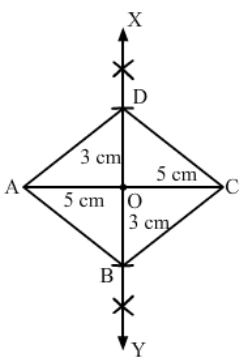1. Draw AC equal to 10 cm.

2. Draw XY, the right bisector of AC, meeting it at O.

3. With O as centre and radius equal to half of the length of the other diagonal, i.e. 3 cm, cut OB = OD = 3 cm.

4. Join AB, AD and CB, CD.

Question: 8

Draw a rhombus, having each side of length 3.5 cm and one of the angles as 40°.

Solution: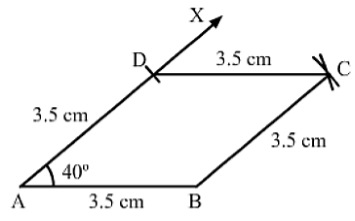1. Draw a line segment AB of 3.5 cm.

2. Draw ∠BAX equal to 40°.

3. With A as the center and the radius equal to AB, cut AD at 3.5 cm.

4. With D as the center, cut an arc of radius 3.5 cm.

5. With B as the centre, cut an arc of radius 3.5 cm. This arc cuts the arc of step 4 at C.

6. Join DC and BC.

Question: 9

One side of a rhombus is of length 4 cm and the length of an altitude is 3.2 cm. Draw the rhombus.

Solution:

1.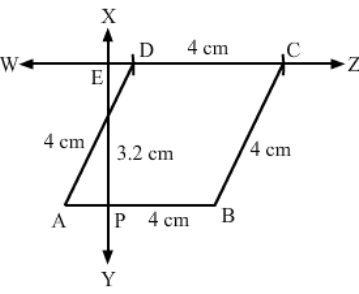Draw a line segment AB of 4 cm.

2. Draw a perpendicular XY on AB, which intersects AB at P.

3. With P as the center, cut PE at 3.2 cm.

4. Draw a line WZ that passes through E. This line should be parallel to AB.

5. With A as the center, draw an arc of radius 4 cm that cuts WZ at D.

6. With D as center and radius 4 cm, cut line DZ. Label it as point C.

Question: 10

Draw a rhombus ABCD, if AB = 6 cm and AC = 5 cm.

Solution: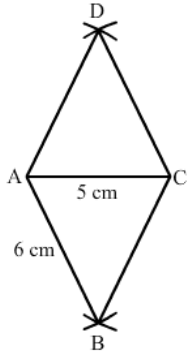1. Draw a line segment AC of 5 cm.

2. With A as centre, draw an arc of radius 6 cm on each side of AC.

3. With C as centre, draw an arc of radius 6 cm on each side of AC. These arcs intersect the arcs of step 2 at B and D.

4. Join AB, AD, CD and CB.

Question: 11

ABCD is a rhombus and its diagonals intersect at 0.

(i) Is ΔBOC = ΔDOC? State the congruence condition used?

(ii) Also state, if ∠ BCO = ∠DCO.

Solution:

(i) Yes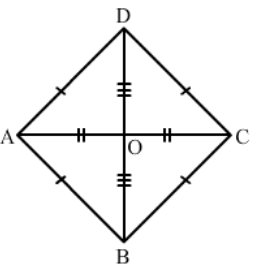In ΔBCO and ΔDCO:

OC = OC (common)

BC = DC (all sides of a rhombus are equal)

BO = OD (diagonals of a rhombus bisect each other

By SSS congruence: ΔBCO ≅ ΔDCO

(ii) Yes

By c.p.c.t:

∠BCO = ∠DCO

Question: 12

Show that each diagonal of a rhombus bisects the angle through which it passes.

Solution:In ΔAED and ΔDEC:

AE = EC (diagonals bisect each other)

AD = DC (sides are equal)

DE = DE (common)

By SSS congruence: ΔAED ≅ ΔCED

Similarly, we can prove ΔAEB and ΔBEC, ΔBEC and ΔDEC, ΔAED and ΔAEB are congruent to each other.

Hence, diagonal of a rhombus bisects the angle through which it passes.

Question: 13

ABCD is a rhombus whose diagonals intersect at 0. If AB = 10 cm, diagonal BD = 16 cm, find the length of diagonal AC.

Solution:

We know that the diagonals of a rhombus bisect each other at right angles.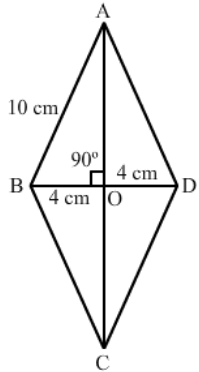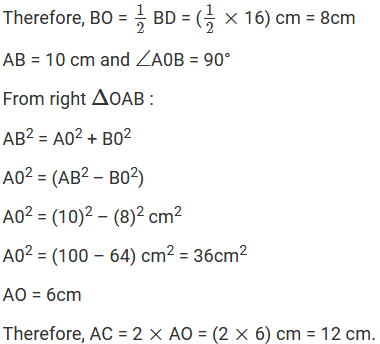Question: 14

The diagonals of a quadrilateral are of lengths 6 cm and 8 cm. If the diagonals bisect each other at right angles, what is the length of each side of the quadrilateral?

Solution: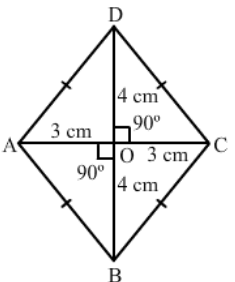Let the given quadrilateral be ABCD in which diagonals AC is equal to 6 cm and BD is equal to 8 cm.

Also, it is given that the diagonals bisect each other at right angle, at point O.

Therefore, AO = OC = (1/2) AC = 3 cm

Also, OB = OD = (1/2) BD = 4 cm

In right ΔAOB:

AB2 = AO2 + BO2

AB2 = (9 + 16) cm2

AB2 = 25 cm2

AB = 5 cm

Thus, the length of each side of the quadrilateral is 5 cm.
```### Course Features

• 728 Video Lectures
• Revision Notes
• Previous Year Papers
• Mind Map
• Study Planner
• NCERT Solutions
• Discussion Forum
• Test paper with Video Solution